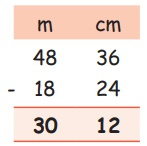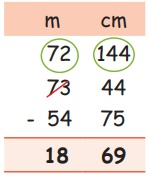Home | | Maths 4th Std | Subtraction of measures

# Subtraction of measures

(i) Subtraction without Regrouping (ii) Subtraction with Regrouping

Subtraction of measures

Subtraction without Regrouping

EXAMPLE

Subtract 18 m 24 cm from 48 m 36 cmStep 1: Subtract centimetre column

(36−24) = 12cm

Step 2: Subtract metre column

48−18 = 30m

Subtraction with Regrouping

EXAMPLE

Subtract 73m 44cm – 54m 75cm75cm cannot be subtracted from 44cm. So take 1m from 73 m and then add with 44 we get 100 + 44 = 144cm.

Step 1: 144cm −75cm = 69cm

Step 2: 72cm −54cm = 18cm

Tags : Measurements | Term 1 Chapter 4 | 4th Maths , 4th Maths : Term 1 Unit 4 : Measurements
Study Material, Lecturing Notes, Assignment, Reference, Wiki description explanation, brief detail
4th Maths : Term 1 Unit 4 : Measurements : Subtraction of measures | Measurements | Term 1 Chapter 4 | 4th Maths